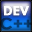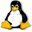Home Latest News Archive Tutorials C++ 2 3 4 Win32 Java OpenGL GLUT OpenGL ES 2 3 4 DirectX VB.NET HTML Photoshop 3dsmax Forums Projects Modelling Links About Us Contact Search Recent Tutorials C / C++ Win32 OpenGL OpenGL ES GLUT## C++ Strings

Tutorials > C++ > C++ Strings

### Introduction

In the previous tutorial, we looked at how to manipulate C style strings. C++ gives you the ability to use a string object. This simplifies tasks greatly as you will see in the following tutorial. Classes will be explained in a future tutorial but for now you will need to know that objects have things called methods. These work the same way as functions and can be accessed in the same way that functions are accessed in structs using the . operator.

A number of methods will be explained for the string class including the following :

length()     find_first_of(char)
find_last_of(char)
find(string)
compare(string)
substr(int, int)
replace(int, int, string)

Contents of main.cpp :

To be able to create string objects, an extra #include needs to be made. The string header must be included and the std. namespace must be used.

```#include <iostream>
#include <stdlib.h>
#include <string>

using namespace std;

int main()
{
```

We can create a string in the same way as a normal character string except we can use the string keyword instead of the char keyword. A string literal can automatically be converted to a string. A string can be printed out as per normal.

```	string str = "Hello World";
cout << str << endl;
```

The length of a string can be found by accessing its length method. Our code therefore returns a value of 11.

```	cout << "String Length : ";
cout << (int)str.length() << endl;
```

No method is needed to append strings onto existing ones. This can be achieved by simply using the + or the += operators.

```	cout << "Appended String : ";
str += ", Bob!";
cout << str << endl;
```

If you would like to find the occurrence of a character, you can use the find_first_of or find_last_of methods depending on if you want to find the first or last occurrence of a character. This method takes one parameter which is the character that you are searching for. An optional integer parameter can be added to specify the offset of the string from where to start searching from. The index of the position found is returned. If the character is not found, a value of -1 is returned.

```	cout << "First position of o : ";
cout << (int)str.find_first_of('o') << endl;
cout << "Last position of o : ";
cout << (int)str.find_last_of('o') << endl;
```

If you would like to search for an entire string, the find method can be used. It works in the exact same way as the find methods above except that a string to search for is passed as the first parameter instead of one character.

```	cout << "World Substring : ";
cout << (int)str.find("World") << endl;
```

To check if two strings are equal you can use the == operator in the same way as other data types.

```	string str1 = "Hello";
string str2 = "Hello";

if (str1 == str2)
cout << "Strings are the same." << endl;
```

Another way of comparing two strings is to use the compare method. The parameter is the string that will be compared with the current string. This provides more useful information and works in the same way as the strcmp function in the previous tutorial. A value of 0 is returned if both strings are equal. A negative value is returned if the current string is less than the given string and the opposite is true for a positive value.

```	if (!str1.compare(str2))
cout << "Strings are the same."
<< endl;
```

A copy of a string's data is easily copied to another string by simply using the = operator.

```	cout << "String Copy : ";
str2 = str1;
cout << str2 << endl;
```

To extract a substring from an original string, the substr method can be used. The first parameter is the start position of the substring and the second parameter is how many characters must be read. The return value is the substring found. In our case, the substring of "World" is returned.

```	cout << "Substring Copy : ";
str1 = str.substr(6, 5);
cout << str1 << endl;
```

Another method you may find useful is the replace method. This method replaces a substring of text within a string with another piece of text. Imagine it being the same as highlighting a piece of text with your mouse and then overwriting it by typing other text. The first parameter is the start position and the second parameter is how many characters to replace. These two parameters essentially specify the highlight. The third parameter is the new string that you want to insert. The code below therefore replaces the "World" part with "Universe".

```	str.replace(6, 5, "Universe");
cout << str << endl;
```

An extremely useful method for the string is the c_str method. This returns the C style string representing the same characters as that of the original string. This allows you to use functions requiring a C style string while still using the easy string class.

```	cout << (int)strlen(str.c_str()) << endl;

system("pause");

return 0;
}```

You should now be proficient in both C and C++ style strings. One can see how C++ strings are more easily manipulated. You should also be able to extract C style strings from C++ strings allowing you to use C++ strings in most situations.

Please let me know of any comments you may have : Contact Me

 Source Files :< Tutorial 32 - Character Strings Tutorial 34 - Recursion >

All Rights Reserved, © Zeus Communication, Multimedia & Development 2004-2005

Read the Disclaimer